×

Search anything:

# Arrays vs Vectors in C++

#### Software Engineering C++Get this book -> Problems on Array: For Interviews and Competitive Programming

The differences between array and vectors in C++ are as follows:

• Array can be static or dynamic; Vector is dynamic
• Array can be traversed using indexes, vector uses iterators
• No reallocation in array
• Size of Array is fixed; Size of vector can be changed
• Vector can be copied using assignment statement
• Vector size is not required when we pass a vector to a function
• Vector can be returned from function; Array cannot be returned
• Arrays are deallocated explicitly; Vectors are deallocated automatically

We have explored the points in depth with C++ code examples.

1. An array can be defined as a collection of elements of same data type. Arrays can be implemented statically or dynamically. For static implementation, while initialising the array one needs to specify the number of elements at an earlier stage, but for dynamic implementation, one can use a pointer of the primitive data type and point to the first element and then ask for the contiguous blocks required. New operator is used for dynamic memory allocation. Whereas vector is a template class implemented as a dynamic array. Here, the vector is a sequential container which uses list interface, as one can easily add and remove elements using push_back() and pop_back() repectively.

Example: The below code shows how arrays can be implemented statically and dynamically. It also shows vector implementation.

``````#include <bits/stdc++.h>
using namespace std;

int main(){
vector<int> myvec; // Vector
int static_arr; // Static Array
int* dy_arr = new int; // Dynamic Array
return 0;
}
``````

1. Elements of an array can be traversed and accessed using numerical indices. A specific element can be accessed using it's index position in the array. In vectors, iterators are used to access any element or just to traverse all the elements present in the vector.

Example:The below code shows how the elements of an array are accessed.

``````#include<bits/stdc++.h>
using namespace std;
int main () {

// nest is an array containing 6 elements
int nest[]={ 2, 4, 6, 8, 10, 12};

cout << "Element \t Value" << endl;

// accessing each element of the array
for ( int j = 0; j < 6 ; j++ ) {
cout << " " << j+1 <<"  \t\t  " << nest[ j ] << endl;
}

return 0;
}
``````

Output: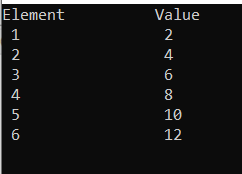Example:The below code shows how the elements of a vector are accessed.

``````#include<bits/stdc++.h>
using namespace std;
int main()
{
vector<int> ar ;
ar.push_back(2);
ar.push_back(4);
ar.push_back(6);
ar.push_back(8);

// Declaring iterator to a vector
vector<int>::iterator ptr;

// Displaying vector elements
cout << "The vector elements are : ";
for (ptr = ar.begin(); ptr < ar.end(); ptr++)
cout << *ptr << " ";

return 0;
}
``````

Output: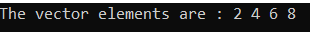1. No new elements can be inserted in the array if the array becomes full because no reallocation is done implicitly in array whereas in the case of vectors, memory is reallocated implicitly and new elements are inserted in the vector.

2. Size of an array is fixed whereas size of a vector is modifiable. It means vector can be resized according to the requirement as they are allocated on heap memory.

Example:The below code shows how the vector resizes itself after a few operations are performed on it.

``````#include <bits/stdc++.h>
using namespace std;

int main(){

vector<int> myVec;

// Inserting Values in Vector
myVec.push_back(1);
myVec.push_back(2);
myVec.push_back(3);
myVec.push_back(4);
myVec.push_back(5);

cout << "Size of vector Before Removal : " << myVec.size() << endl;

//  Removing two elements from the vector
myVec.pop_back();
myVec.pop_back();

cout << "Size of vector After Removal : " << myVec.size() << endl;

return 0;
}
``````

Output: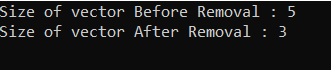1. In order to create a duplicate array of the original one, we have to follow the iterative method i.e running a loop to copy each element at respective index. Whereas, vectors can be copied or assigned directly using the assignment operator.

Example:The below code shows how vectors can be assigned directly using the assignment operator.

``````#include <bits/stdc++.h>
using namespace std;

int main()
{
vector<int> vec;
vector<int> dup_vec;

vec.push_back(10);
vec.push_back(100);
vec.push_back(1000);
vec.push_back(10000);

cout << "Contents of vector (vec):\n";
for( int i=0; i<vec.size(); i++){
cout<<vec[i]<<" ";
}

dup_vec = vec; // Copying vec into dup_vec

cout << "\n\nContents of vector (dup_vec):\n";
for( int i=0; i<dup_vec.size(); i++){
cout<<dup_vec[i]<<" ";
}

return 0;
}
``````

Output: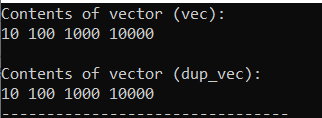1. Vector size is not required when we pass a vector to a function. This is because vector maintains variables which keeps track of size of container at all times. But when arrays are passed to a function, a separate parameter for size is also passed to the function.

2. C++ does not allows to return the address of a local variable to outside of the function so you would have to define the local variable as static variable. Whereas vectors can be returned from a function.

Example: The code below shows vectors can be returned from a function.

``````#include <bits/stdc++.h>
using namespace std;

// Function returning vector
vector<int> demo_function() {

vector<int> ans;
int i=5;
while(i>0){
//inserting values in vector
ans.push_back(i);
i--;
}
return ans;

}

int main() {

vector<int> result;

result = demo_function(); // Call function

// Output Values of vector
cout<<"Vector consists of elements : \n";
for (int i=0; i<result.size();i++)
cout << result[i] << " ";

return 0;
}
``````

Output: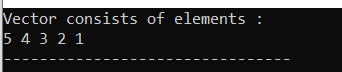1. We can always get the size of the vector in O(1) time using the size() function. But in case of dynamic arrays, the size of array cannot be determined.

2. Dynamic arrays need to be deallocated explicitly whereas vectors are automatically de-allocated from heap memory as soon as variable goes out of scope.

Example:

``````#include <bits/stdc++.h>
using namespace std;

int main()
{
int* arr_demo = new int; // Dynamic Array
vector<int> v; /* Vector is deallocated implicitly
when the program terminates */

delete[] arr_demo; /* Array needs to be deallocated
explicitly before program terminates */

return 0;
}
``````

## Question

#### WHICH ONE IS MORE MEMORY EFFICIENT, ARRAYS OR VECTORS?

Arrays
Vectors
Vector occupies much more memory in exchange for the ability to manage storage and grow dynamically whereas Arrays are memory efficient data structure.

With this article at OpenGenus, you must have gained a good idea of Arrays V/s vectors in C++.